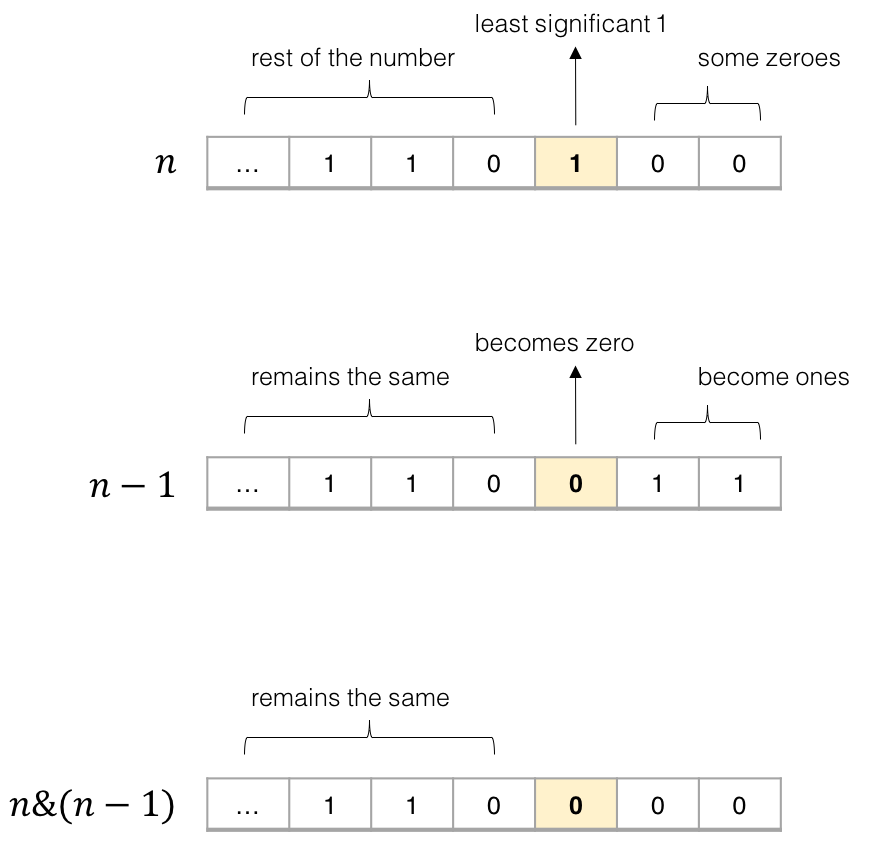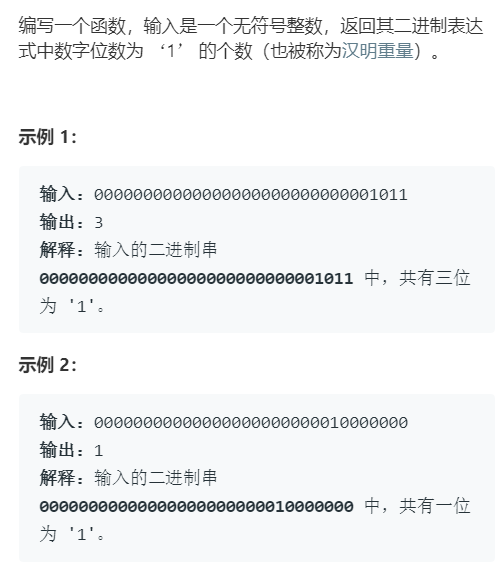### 一、几个有趣的位操作

1. 利用或操作 | 和空格将英文字符转换为小写
('a' | ' ') = 'a'
('A' | ' ') = 'a'

1. 利用与操作 & 和下划线将英文字符转换为大写
('b' & '_') = 'B'
('B' & '_') = 'B'

1. 利用异或操作 ^ 和空格进行英文字符大小写互换
('d' ^ ' ') = 'D'
('D' ^ ' ') = 'd'


PS：以上操作能够产生奇特效果的原因在于 ASCII 编码。字符其实就是数字，恰巧这些字符对应的数字通过位运算就能得到正确的结果，有兴趣的读者可以查 ASCII 码表自己算算，本文就不展开讲了。

1. 判断两个数是否异号
int x = -1, y = 2;
bool f = ((x ^ y) < 0); // true

int x = 3, y = 2;
bool f = ((x ^ y) < 0); // false


PS：这个技巧还是很实用的，利用的是补码编码的符号位。如果不用位运算来判断是否异号，需要使用 if else 分支，还挺麻烦的。读者可能想利用乘积或者商来判断两个数是否异号，但是这种处理方式可能造成溢出，从而出现错误。

1. 交换两个数
int a = 1, b = 2;
a ^= b;
b ^= a;
a ^= b;
// 现在 a = 2, b = 1

1. 加一
int n = 1;
n = -~n;
// 现在 n = 2

1. 减一
int n = 2;
n = ~-n;
// 现在 n = 1


PS：上面这三个操作就纯属装逼用的，没啥实际用处，大家了解了解乐呵一下就行。

### 二、算法常用操作 $n\ \&\ (n-1)$1. 计算汉明权重（Hamming Weight）int hammingWeight(uint32_t n) {
int res = 0;
while (n != 0) {
n = n & (n - 1);
res++;
}
return res;
}

1. 判断一个数是不是 $2$ 的指数

2^0 = 1 = 0b0001
2^1 = 2 = 0b0010
2^2 = 4 = 0b0100


bool isPowerOfTwo(int n) {
if (n <= 0) return false;
return (n & (n - 1)) == 0;
}


40

5

2